THE FAMILY OF METALLIC MEANS

Centro de Matemática y Diseño -Facultad de Arquitectura, Diseño y Urbanismo

José María Paz 1131 – Florida (1602) – Buenos Aires – Argentina

Postmaster@caos.uba.ar

1. INTRODUCTION

Let me introduce you to the Metallic Means Family (MMF). Their members have, among other common characteristics, the property of carrying the name of a metal. Like the very well known Golden Mean and its relatives, the Silver Mean, the Bronze Mean, the Copper Mean, the Nickel Mean and many others. The Golden Mean has been used in a very big number of ancient cultures as a proportion basis to compose music, devise sculptures and paintings or construct temples and palaces (see Reference ). Some of the relatives of the Golden Mean have been used by physicists in their latest researches trying to analyze the behavior of non-linear dynamical systems in going from periodicity to quasi-periodicity ( and ). But in quite a different context, Jay Kappraff  uses the Silver Mean to describe and explain the roman system of proportions, referring to a mathematical property that, as we shall prove, it is common to all the members of this remarkable family.

2. FIBONACCI SEQUENCES

The Fibonacci sequence is a sequence of integer numbers, where every number is the sum of the two previous ones. Such sequences are called "secondary Fibonacci sequences" to distinguish them from the tertiary Fibonacci sequences, were every term is the sum of the three previous terms. Beginning with F (0) = 1; F (1) = 1, we get

(2.1)

1, 1, 2, 3, 5, 8, 13, 21, 34, 55, 89, 144,...

where

F (n + 1) = F (n) + F (n - 1).

The secondary Fibonacci sequences may be generalized, originating the so called "generalized secondary Fibonacci sequence" GSFS, a, b, pb + qa, p(pb + qa) + qb, that satisfy relations of the type

(2.2)

G (n+1) = p G (n) + q G (n - 1)

p and q are natural numbers. From (2.2) we getTaking limits in both members and assuming thatexists and is equal to a real number x – as will be proved in next theorem -- we haveor else x2 - px - q = 0, which positive solution isThis means

(2.3)THEOREM 1.

Given a GSFS a, b, pb + qa, p(pb + qa) + qb, ... such that G(n+1) = p G(n) + q G(n-1), with p,q natural numbers, then there existsand it is a real positive number.

Proof: to find an expression for the nth term of the GSFS, let us write equation (2.2) in the form G(n+1) = p G(n) + qH(n), where H(n+1) = G(n). This last equation can be written in matricial from introducing the matricesThen it is easy to prove that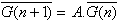or else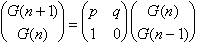Let us assume G(0) = G(1) =1 for simplicity. Ifthenand the problem is reduced to the finding of the nth power of matrix A. The characteristic equation of A iswith the two eigenvaluesTo diagonalize A and convert it inwe shall use the change of base matrixThe nth power of A is calculated applying the similarity transformation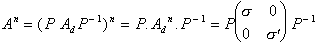Finally we haveso thatReplacing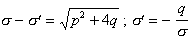we getand the proof is complete for the sequence: 1, 1, p + q, p(p + q) + q,...

If, instead of choosing G(0) = G(1) = 1 we begin with two arbitrary values a and b, it is easy to prove that the result is the same. In fact, given the GSFS: a, b, pb + qa, p(pb + qa) + qb, ... we have to evaluate the ratio3. THE FAMILY OF METALLIC MEANS

Let us consider a set of positive irrational numbers, obtained taking G(0) = G(1) = 1 in equation (2.3) and considering different values for the parameters p and q.

Definition:The metallic means family (MMF) is the set of positive eigenvalues of the matricial equation

(3.1)for different values of p and q (natural numbers).

All the members of this family are positive quadratic irrational numbers that are the positive solutions of quadratic equations of the type

(3.2)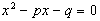Let us begin withThen it is very easy to find the members of the MMF that satisfy this equation, expanding them in continued fractions. In fact, if p = q = 1, it results x2 = x + 1, that can be written:Replacing iteratively the value of x of the second term, we havethat is

x = [1,1,1, ...] =a purely periodic continued fraction that defines the Golden Mean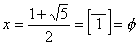Analogously, if p = 2 y q = 1 we obtain the Silver Meananother purely periodic continued fraction.

If p = 3 and q = 1, we get the Bronze MeanFor p = 4; q = 1, the metallic meanis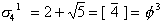a striking result related to the continued fraction expansion of uneven powers of the Golden Mean. The remaining metallic means are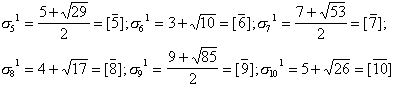Obviously, all of them are of the form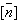, a purely periodic continued fraction expansion. The slowest converging one of all them is the Golden Mean, since all its denominators are the smallest possible (ones). An elegant way of stating this result is

The Golden Mean f is the most irrational of all irrational numbers.we have for q = 1, again the Golden Mean. If p = 1 and q = 2, we obtain the Copper Meana periodic continued fraction. If p = 1 and q = 3, we get the Nickel Mean,Analogously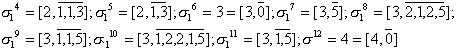and all these members of the MMF are of the formFurthermore, it is very easy to verify that in this set, the integer metallic means,,,..., appear in quite a regular way. The first for q = 2 (1+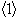=2), the second for q = 6 (3+=6), the third for q = 12 (7+=12), the forth for q = 20 (13+=20), the fifth for q = 30 (21+=30), and so on, where <.> is the number of equations separating two consecutive integer solutions. The first digit of the metallic mean that is not equal to an integer is m = 2 for the first three; m = 3 for the second five; m = 4 for the third seven; m = 5 for the fourth nine, etc. The continued fraction expansions of the non integer metallic means are "palindromic", that is, the periods are symmetrical with respect to their centers, with the exception of the last digit of the period that is equal to 2m-1. There is no simple rule which predicts the length of the periods, some of them are very short like for example, the first after an integer solution has a period equal to 1:

q = 3 (); q = 7 (); q = 13 (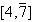); q = 21 (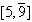); ...

and the last ones before an integer solution are of the form:

q = 5 (); q = 11 (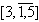); q = 19 (); ...,

that is, they have periods of length 2. Other periods are extremely long and with the exception of the cases q = 3,7,13,21,31,... the remaining continued fraction expansions present "stable cycles" of different lengths.

Let us consider the sequence of ratios of consecutive terms of the sequence (2.1). The result is a new sequencethat converges to the Golden Mean f . If we take now a geometric progression of ratio f:it is easy to verify that this geometric progression is also a GSFS that satisfies relation (2.2). In fact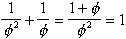Similarly for the Silver Mean s Ag. Starting from the sequence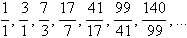that converges to s Ag, it is easy to prove that the sequenceis a geometric progression of ratio s Ag that satisfies A(n+2) = 2 A(n+ 1) + A(n).

Indeed .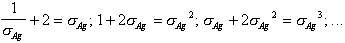In an analogous way, it is proved that the sequenceconverges to the Bronze Mean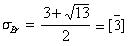and originates a geometric progression of ratio sBr. that satisfies B(n + 2) = 3 B(n + 1) + B(n).

The same happens for all the GSFS that satisfy condition (2.3) and this fact allows us to state the following unique mathematical property:

The members of the MMF are the only positive quadratic irrational numbers that originate GSFS (with additive properties), which are, simultaneously, geometric progressions.

This curious property of satisfying both arithmetic additive and geometric properties, bestow all the members of the MMF interesting characteristics to become basis of different systems of geometric proportions in Design.

5. PROPORTIONAL SYSTEMS

The Golden Mean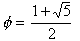is linked to pentagonal symmetry. Indeed, if we consider a pentagon of unity side (Fig. 5.1), it is easy to verify that its diagonal is equal to f and besides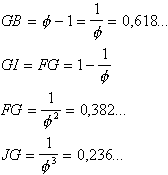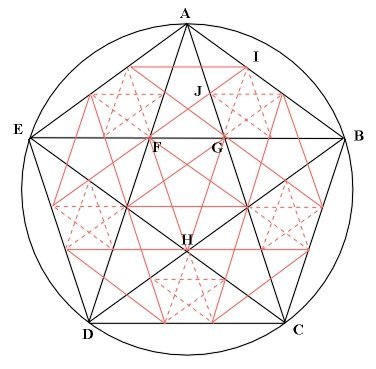Figure 5.1

These Golden divisions determine the proportions of the beautiful ancient Hermes mask (Medusa) shown in Fig. 5.2. It is a Roman relief in marble reproduced from the Greek original, 1st century BC. that is exhibited at the Glyptothek, Munich, Germany.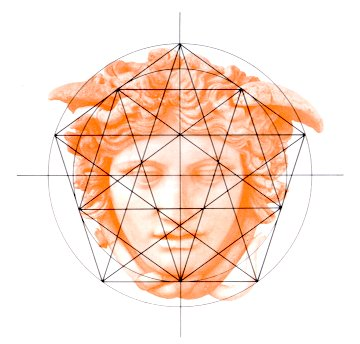Figure 5.2

Innumerable are the references to the apparition of the Golden Mean f in the proportional systems adopted by ancient civilizations in their constructions as well as its presence in the proportions of the human body and in botany. Among the many authors who have dedicated their investigations to this subject we have to mention Matila Ghyka ,  and , H. E. Huntley  and Theodore Andrea Cook, whose book , published in 1979, is a reprint of the original published by Constable, London, England in 1914.

More recently, Jay Kappraff , at the conference Nexus’96: Relations between Architecture and Mathematics, that was held at Fucecchio (near Florence) in June 1996, carried out a careful analysis of the three architectonic proportional systems presented by P. H. Scholfield in his excellent book . The three proportional systems are: 1) the system of musical proportions used during the Italian Renaissance, developed by León Battista Alberti ; 2) the Modulor created by Le Corbusier  and 3) the Roman proportional system.

The musical system was based on rational proportions inherent in the musical scale. Although it succeeded in creating harmonic relationships in which key proportions were repeated in a design, it did not have the additive properties necessary for a successful proportional system. The Modulor of Le Corbusier is based on the Golden Mean f (see References ,), while the Roman system of proportions is based on the Silver Mean. And both conform to the relationships inherent in the system of musical proportions, with the great advantage of having additive properties.

Donald and Carol Watts , a married couple of American architects, have carefully studied the ruins of the Garden Houses at Ostia, the city-port of the Roman empire and they found that all these houses have been designed using theoretically the roman system of proportions . These are not the only examples from antiquity in which the Silver Mean is present; similar results have been found by Kim Williams in the pavement of the baptistery of San Giovanni , Verrocchio’s Tombslab for Cosimo de’Medici  and Michaelangelo’s famous Medici Chapel in Florence .

6. ST. GEORGE FRACTAL SCULPTURES

Alan St. George is a retired architect, of British origin, who lives in Portugal and is dedicated to the creation of mathematical sculptures. In December 1995 he presented in Lisbon his exhibition "The form of the number" . His originals are acrylic or metallic plates and can be reproduced using computer graphs. The generation of these original sculptures is based on the fractal principle  of adding to each of the five platonic solids reduced versions of the same solid. For example, to convert a cube in a fractal octahedron, one begins with a cube which faces are divided in nine equal squares (Fig. 6.1). Then a cross with six small cubes which faces are the mentioned squares, is constructed. Five of these cubes are disposed in "Greek cross" and the sixth is put over the central cube, forming a sort of stacked pyramid. The process goes on by sticking one of these pyramids on each of the six faces of the original cube. Then, each of the faces of the resultant structure is divided into nine smaller squares on which reduced copies of the pyramid are sticked.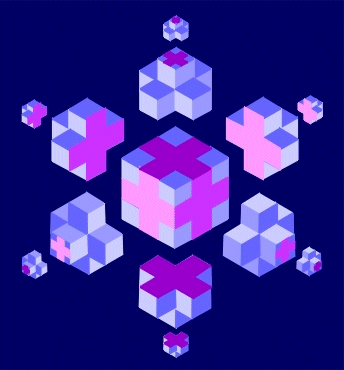Figure 6.1

It is also possible to fractalize an octahedron arriving to a tetrahedron or a cube (Fig. 6.2), like it is indicated by the mathematician Ian Stewart . And why not? We could even imagine the application of this fractalization process to half-regular solids of pentagonal symmetry that include the Golden Mean in its characteristics or to those of octagonal symmetry that include the Silver Mean.

Another variant of St. George’s sculptures consists in constructing three-dimensional spirals, starting with the five platonic solids. In particular, let us consider the icosahedron of pentagonal symmetry, which main characteristics are:

Faces: 20 Vertices: 12 Edges: 30

Length of edge: 1

Center of polyhedron to center of face:= 0,7558

Center of polyhedron to mid-edge: f /2 = 0,8090...

Center of polyhedron to vertex:= 0,9511...

Volume:= 2,1817...Figure 6.2

It is then easy to construct an "icosahedrical spiral" (Fig. 6.3a) following a path that runs through the twelve triangular edges of the icosahedron, touching each vertex only once. If we consider an ant that begins to walk at vertex A (Fig. 6.3b,c), we see an icosahedron. The construction is realized by a sequence of "legs" that correspond to the 12 edges of the ant's path. Each leg is connected to the previous one and runs parallel to an edge. But successive legs have different lengths:= 1,040916... times the length of its predecessor. The answer to the question: why such a strange figure?, is that after having added 12 edges to a given one, the last of them runs parallel to the original, having increased its size in ()12 = f .Figure 6.3aFigure 6.3b,c

Obviously, the election of the Golden Mean f in the construction of St. George’s icosahedrical spiral, obeys to aesthetic reasons. Nevertheless, it is impossible to deny the mathematical reality of a pentagonal symmetry intrinsically linked to the Golden Mean ...

7. PISOT AND SALEM NUMBERS

Let us consider the set U of real algebraic integers greater than 1, which are solution to the irreducible polynomial of the form xm = am-1xm-1 + ... + a1x + a0 (ai are integers) such that all other solutions (Galois conjugates) have modulus at most equal to 1. This set is divided into two disjoint sets, S and T. The set S of Pisot numbers (introduced by Charles Pisot, 1910-1984 , in his famous thesis published in 1938) or else Pisot-Vijayaraghavan’s numbers, is the set of real algebraic integers q > 1 whose other conjugates have modulus strictly smaller than 1. The set T of Salem numbers (discovered by Raphael Salem, 1898-1963) is the set of real algebraic integers t > 1 whose other conjugates have modulus at most equal to 1, one at least having a modulus equal to 1 .

The sets S and T define a partition of U. All rational numbers greater than 1 belong to S. The quadratic numbers in S are zeros of second degree polynomials with integer coefficients x2 + q1x + q0 with q1 + |1 + q0| < 0. It is easy to prove that S is a closed set on the real line. The set of limit points of S is called the "derived set of S" and is denoted by S’ Furthermore, if q is a Pisot number, then q m Î S’ for every integer m ³ 2. This implies that all Pisot numbers of degree 2 Î S’, being the smallest of them the Golden Mean ,. which is also the least element of S’.

Quite recently, it has been discovered , , , , that Pisot numbers are natural candidates for coordinating quasicrystalline nodes in 1, 2 or 3 dimensions, and also the Bragg peaks in related diffraction patterns. In the observed cases, the following Pisot numbers appear as self-similarity ratios in quasicrystalline structures:The first figure (the well known Golden Mean) corresponds to penta or decagonal quasilattices. The second one (the Silver Mean) corresponds to the octagonal case and the third one, to the dodecagonal case. Certainly, the metallic means which expansion in continued fractions is purely periodic are quadratic Pisot numbers because they are the positive solution of quadratic equations of the formp natural number.

Besides, the positive solutions of quadratic equations of the type x2px + 1 = 0, whereare also quadratic Pisot numbers with purely periodic continued fraction expansions, where the condition that the terms of the continued fraction have to be positive, has been relaxed . For example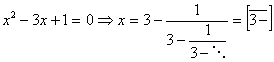.

On the contrary, there are no examples of Salem numbers as simple as the ones given for Pisot numbers because there exist no Salem numbers of degree less than 4. Notwithstanding, both sets of Pisot numbers and of Salem numbers have many important applications not only in the quasicrystalline context, where they could play the same role as ordinary integers do in crystallography, but also in the formal study of power series and harmonic analysis.

8. CONCLUSIONS

The members of the MMF are intrinsically related with the onset from a periodic dynamics to a quasi-periodic dynamics, with the transition from order to chaos and with time irreversibility, as proved by Ilya Prigogine  and M. S. El Naschie .

But, simultaneously, there are philosophical, natural and aesthetically considerations that have impelled the utilization of proportions based on some members of the MMF, from the beginning of human history. They appeared in the Egyptian sacred art as well as in India, China, Islam and many other ancient civilizations. They have dominated the Greek art and architecture, they extended to the magnificent monuments of the Gothic Middle Age and they reappeared with all its splendor in the Renaissance period.

In many instances, the harmony and beauty of a pattern is the result of the influence of the Golden and Silver Means at a fundamental level.

Such a wide range of applications where the members of the MMF appear, opens many roads to new inter-disciplinary investigations that will undoubtedly clear up the existent relations between Art and Technology, establishing a bridge among the rational scientific approach and the esthetical emotion. And perhaps, this new perspective could help us to give to Technology, from which we depend increasingly for our survivorship, a more human aspect.

REFERENCES

 Vera W. de Spinadel, From the Golden Mean to Chaos, Nueva Librería, 1998.

 Vera W. de Spinadel, On characterization of the onset to chaos. Chaos, Solitons and Fractals, vol. 8, Nr 10, pp. 1631-1643, 1997.

 J. Kappraff, Musical proportions at the basis of systems of architectural proportion both ancient and modern. In NEXUS - Architecture and Mathematics. Ed.: Kim Williams, 1996.

 Matila C. Ghyka, Estética de las proporciones en la naturaleza y en las artes. Editorial Poseidón, S. L., Barcelona,1977.

 Matila C. Ghyka, El número de oro, 2 volumes. Editorial Poseidón, S. L., Barcelona, 1978.

 Matila C. Ghyka, The Geometry of Art and Life, Dover Publications Inc., New York, 1977.

 H. E. Huntley, The Divine Proportion - A study in mathematical beauty, Dover Publications Inc., New York, 1970.

 Theodore Andrea Cook, The curves of life, Dover Publications Inc., New York, 1979.

 P. H. Scholfield, The theory of proportion in Architecture, Cambridge: Cambridge University Press, 1958.

 León Battista Alberti, The ten books of Architecture, 1755, reprint by Dover Publications Inc., New York, 1986.

 Le Corbusier, Le Modulor, 1950. Spanish translation by Rosario Vera, El Modulor: Ensayo sobre una medida armónica a la escala humana aplicable universalmente a la arquitectura y a la mecánica, Buenos Aires: Poseidón, 1953.

1954. Modulor 2, Paris. Spanish translation by Albert Junyent, Modulor 2 (Los usuarios tienen la palabra). Continuación de El Modulor 1948. Buenos Aires: Poseidón 1962.

 Vera W. de Spinadel, El Modulor de Le Corbusier, AREA Agenda de reflexión en Arquitectura, Diseño y Urbanismo, No. 3, February 1996.

 Vera W. de Spinadel, Algo más de Matemática, AREA Agenda de reflexión en Arquitectura, Diseño y Urbanismo, No. 4, August 1996.

 Donald J. Watts y Carol M. Watts, A roman apartment complex, Scientific American, vol. 255, No. 6, December 1986.

 Vera W. de Spinadel, The Metallic Means and design. In NEXUS II- Architecture and Mathematics. Ed.: Kim Williams, 1998.

 Kim Williams, The Sacred Cut revisited: the pavement of the Baptistry of San Giovanni, Florence, The Mathematical Intelligencer, vol. 16, No. 2, September 1994.

 Kim Williams, Verrocchio’s Tombslab for Cosimo de’Medici: designing with a mathematical vocabulary. In NEXUS - Architecture and Mathematics. Ed. Kim Williams, 1996.

 Kim Williams, Michelangelo’s Medici Chapel: the cube, the square and the Ö 2 rectangle. Leonardo, vol. 30, No. 2, pp. 105-112, 1997.

 Ian Stewart, Las esculturas de Alan St. George, Investigación y Ciencia, July 1996.

 Vera de Spinadel, Jorge G. Perera and Jorge H. Perera, Geometría fractal, Nueva Librería, 1993, 1994.

 Ian Stewart, Cuentos de un número desdeñado, Investigación y Ciencia, August 1996.

 M. J. Bertin, A. Decomps-Guilloux, M. Grandet-Hugot, M. Pathiaux-Delefosse and J. P. Schreiber, Pisot and Salem Numbers, Birkhäuser, 1992.

 Damien Barache, Bernard Champagne and Jean-Pierre Gazeau, Pisot-cyclotomic quasilattices and their symmetry semigroups. In Quasicrystals and discrete Geometry, Fields Institute Monographs, editor Jirí Patera, American Mathematical Society, 1998.

 J.-P. Gazeau, Pisot-cyclotomic integers for quasilattices, R. V. Moody (ed.), The Mathematics of long-range aperiodic Order, 175-198, 1995.

 C. Burdik, Ch. Frougny, J.-P. Gazeau and R. Krejcar, Beta-integers as natural counting systems for quasicrystals, J. Phys. A: Math. Gen. vol. 31, 6449-6472, 1998.

 J.-P. Gazeau, Counting systems with irrational basis for quasicrystals, to appear in Les Houches School Proceedings.

 Vera W. de Spinadel, "Triangulature" in Andrea Palladio, Nexus Network Journal, Architecture and Mathematics on line, ed. Kim Williams, vol. 1, No. 1, January 1999.

 Ilya Prigogine, El fin de las certidumbres, Andrés Bello, Chile, 1996.

 M. S. El Naschie and I. Prigogine, Time symmetry breaking in Classical and Quantum Mechanics, Chaos, Solitons and Fractals, vol. 7 (4), Special Issue, 1996.